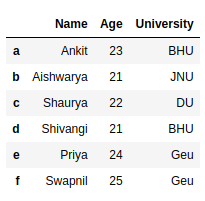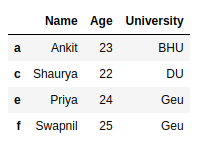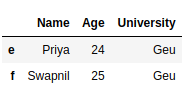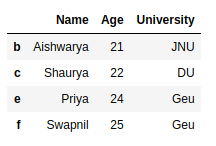# How to Drop rows in DataFrame by conditions on column values?

• Last Updated : 02 Jul, 2020

In this article, we are going to see several examples of how to drop rows from the dataframe based on certain conditions applied on a column.

Pandas provide data analysts a way to delete and filter data frame using `dataframe.drop()` method. We can use this method to drop such rows that do not satisfy the given conditions.

Let’s create a Pandas dataframe.

 `# import pandas library ` `import` `pandas as pd ` ` `  `# dictionary with list object in values ` `details ``=` `{ ` `    ``'Name'` `: [``'Ankit'``, ``'Aishwarya'``, ``'Shaurya'``, ` `              ``'Shivangi'``, ``'Priya'``, ``'Swapnil'``], ` `    ``'Age'` `: [``23``, ``21``, ``22``, ``21``, ``24``, ``25``], ` `    ``'University'` `: [``'BHU'``, ``'JNU'``, ``'DU'``, ``'BHU'``,  ` `                    ``'Geu'``, ``'Geu'``], ` `} ` ` `  `# creating a Dataframe object  ` `df ``=` `pd.DataFrame(details, columns ``=` `[``'Name'``, ``'Age'``, ` `                                      ``'University'``], ` `                  ``index ``=` `[``'a'``, ``'b'``, ``'c'``, ``'d'``, ``'e'``, ` `                           ``'f'``]) ` ` `  `df `

Output:Example 1 : Delete rows based on condition on a column.

 `# import pandas library ` `import` `pandas as pd ` ` `  `# dictionary with list object in values ` `details ``=` `{ ` `    ``'Name'` `: [``'Ankit'``, ``'Aishwarya'``, ``'Shaurya'``, ` `              ``'Shivangi'``, ``'Priya'``, ``'Swapnil'``], ` `    ``'Age'` `: [``23``, ``21``, ``22``, ``21``, ``24``, ``25``], ` `    ``'University'` `: [``'BHU'``, ``'JNU'``, ``'DU'``, ``'BHU'``,  ` `                    ``'Geu'``, ``'Geu'``], ` `} ` ` `  `# creating a Dataframe object  ` `df ``=` `pd.DataFrame(details, columns ``=` `[``'Name'``, ``'Age'``, ` `                                      ``'University'``], ` `                  ``index ``=` `[``'a'``, ``'b'``, ``'c'``, ``'d'``, ``'e'``, ``'f'``]) ` ` `  `# get names of indexes for which ` `# column Age has value 21 ` `index_names ``=` `df[ df[``'Age'``] ``=``=` `21` `].index ` ` `  `# drop these row indexes ` `# from dataFrame ` `df.drop(index_names, inplace ``=` `True``) ` ` `  `df `

Output :Example 2 : Delete rows based on multiple conditions on a column.

 `# import pandas library ` `import` `pandas as pd ` ` `  `# dictionary with list object in values ` `details ``=` `{ ` `    ``'Name'` `: [``'Ankit'``, ``'Aishwarya'``, ``'Shaurya'``,  ` `              ``'Shivangi'``, ``'Priya'``, ``'Swapnil'``], ` `    ``'Age'` `: [``23``, ``21``, ``22``, ``21``, ``24``, ``25``], ` `    ``'University'` `: [``'BHU'``, ``'JNU'``, ``'DU'``, ``'BHU'``, ` `                    ``'Geu'``, ``'Geu'``], ` `} ` ` `  `# creating a Dataframe object  ` `df ``=` `pd.DataFrame(details, columns ``=` `[``'Name'``, ``'Age'``, ` `                                      ``'University'``], ` `                  ``index ``=` `[``'a'``, ``'b'``, ``'c'``, ``'d'``, ``'e'``, ``'f'``]) ` ` `  `# get names of indexes for which column Age has value >= 21 ` `# and <= 23 ` `index_names ``=` `df[ (df[``'Age'``] >``=` `21``) & (df[``'Age'``] <``=` `23``)].index ` ` `  `# drop these given row ` `# indexes from dataFrame ` `df.drop(index_names, inplace ``=` `True``) ` ` `  `df `

Output :Example 3 : Delete rows based on multiple conditions on different columns.

 `# import pandas library ` `import` `pandas as pd ` ` `  `# dictionary with list object in values ` `details ``=` `{ ` `    ``'Name'` `: [``'Ankit'``, ``'Aishwarya'``, ``'Shaurya'``, ` `              ``'Shivangi'``, ``'Priya'``, ``'Swapnil'``], ` `    ``'Age'` `: [``23``, ``21``, ``22``, ``21``, ``24``, ``25``], ` `    ``'University'` `: [``'BHU'``, ``'JNU'``, ``'DU'``, ``'BHU'``,  ` `                    ``'Geu'``, ``'Geu'``], ` `} ` ` `  `# creating a Dataframe object  ` `df ``=` `pd.DataFrame(details, columns ``=` `[``'Name'``, ``'Age'``, ` `                                      ``'University'``], ` `                  ``index ``=` `[``'a'``, ``'b'``, ``'c'``, ``'d'``, ``'e'``, ``'f'``]) ` ` `  `# get names of indexes for which ` `# column Age has value >= 21 ` `# and column University is BHU ` `index_names ``=` `df[ (df[``'Age'``] >``=` `21``) & (df[``'University'``] ``=``=` `'BHU'``)].index ` ` `  `# drop these given row ` `# indexes from dataFrame ` `df.drop(index_names, inplace ``=` `True``) ` ` `  `df `

Output :My Personal Notes arrow_drop_up
Recommended Articles
Page :